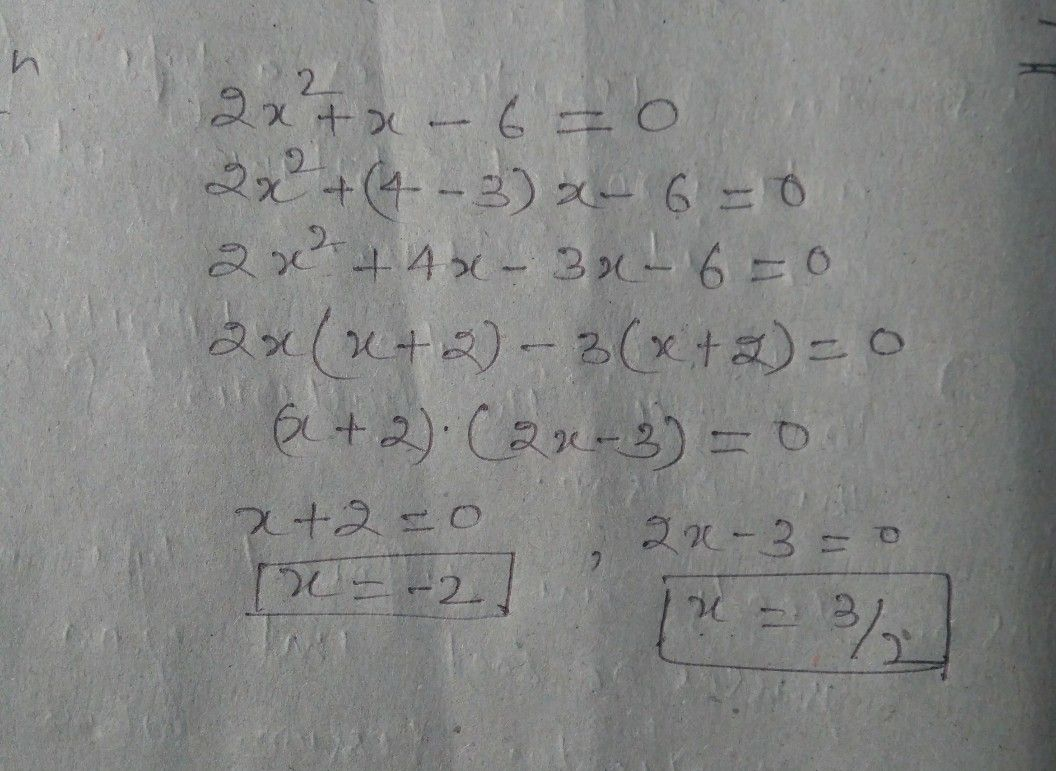Symbol
Problema $.$ $\dfrac {x} {5}$ $2x^{2}+x-6=0$ Solve the equation: $2x^{2}+x-6=0$